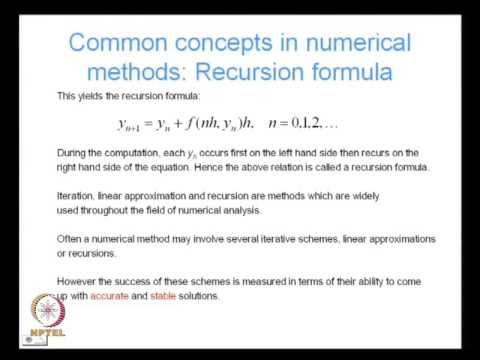### Numerical Methods in CivilIntroduction to Numerical Methods:
• Why study numerical methods.
• Sources of error in numerical solutions: truncation error, round off error.
• Order of accuracy - Taylor series expansion.
Direct Solution of Linear systems:
• Gauss elimination, Gauss Jordan elimination.
• Pivoting, inaccuracies due to pivoting.
• Factorization, Cholesky decomposition.
• Diagonal dominance, condition number, ill conditioned matrices, singularity and singular value decomposition.
• Banded matrices, storage schemes for banded matrices, skyline solver.
Iterative solution of Linear systems:
• Jacobi iteration.
• Gauss Seidel iteration.
• Convergence criteria.
Direct Solution of Non Linear systems:
• Newton Raphson iterations to find roots of a 1D nonlinear equation.
• Generalization to multiple dimensions.
• Newton Iterations, Quasi Newton iterations.
• Local and global minimum, rates of convergence, convergence criteria.
Iterative Solution of Non Linear systems:
• Preconditioning.
Partial Differential Equations:
• Introduction to partial differential equations.
• Definitions & classifications of first and second order equations.
• Examples of analytical solutions.
• Method of characteristics.
Numerical Differentiation:
• Difference operators (forward, backward and central difference).
• Stability and accuracy of solutions.
• Application of finite difference operators to solve initial and boundary value problems.
Introduction to the Finite Element Method as a method to solve partial differential equations:
• Strong form of the differential equation.
• Weak form.
• Galerkin method: the finite element approximation.
• Interpolation functions: smoothness, continuity, completeness, Lagrange polynomials.
Numerical integration of time dependent partial differential equations:
• Parabolic equations: algorithms - stability, consistency and convergence, Lax equivalence theorem.
• Hyperbolic equations: algorithms - Newmark's method,stability and accuracy, convergence, multi-step methods.
Numerical solutions of integral equations:
• Types of integral equations.
• Fredholm integral equations of the first and second kind.
• Fredholm's Alternative theorem.
• Collocation and Galerkin methods for solving integral equations.